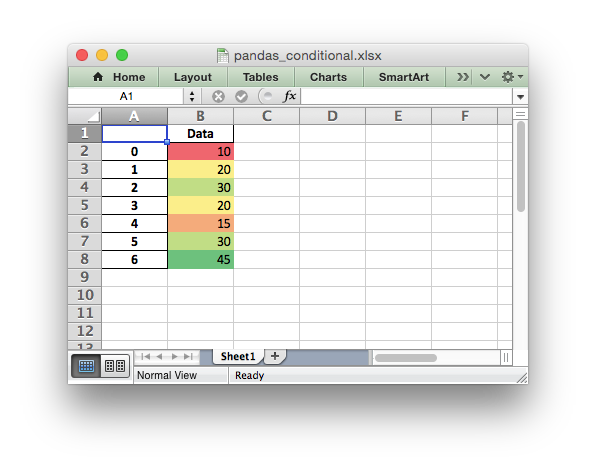# Example: Pandas Excel output with conditional formatting#

An example of converting a Pandas dataframe to an Excel file with a conditional formatting using Pandas and XlsxWriter.```##############################################################################
#
# An example of converting a Pandas dataframe to an xlsx file with a
# conditional formatting using Pandas and XlsxWriter.
#
# Copyright 2013-2023, John McNamara, jmcnamara@cpan.org
#

import pandas as pd

# Create a Pandas dataframe from some data.
df = pd.DataFrame({"Data": [10, 20, 30, 20, 15, 30, 45]})

# Create a Pandas Excel writer using XlsxWriter as the engine.
writer = pd.ExcelWriter("pandas_conditional.xlsx", engine="xlsxwriter")

# Convert the dataframe to an XlsxWriter Excel object.
df.to_excel(writer, sheet_name="Sheet1")

# Get the xlsxwriter workbook and worksheet objects.
workbook = writer.book
worksheet = writer.sheets["Sheet1"]

# Get the dimensions of the dataframe.
(max_row, max_col) = df.shape

# Apply a conditional format to the required cell range.
worksheet.conditional_format(1, max_col, max_row, max_col, {"type": "3_color_scale"})

# Close the Pandas Excel writer and output the Excel file.
writer.close()
```